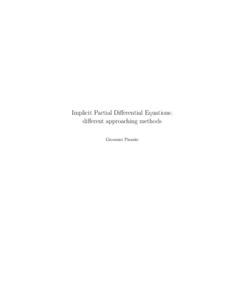Ricerca
Browse
Informazioni

Pisante, Giovanni (2004) Implicit Partial Differential Equations: different approaching methods. [Tesi di dottorato] (Unpublished)Preview
PDF
Pisante-Tesi.pdf

[error in script] [error in script]
Item Type: Tesi di dottorato
Resource language: English
Title: Implicit Partial Differential Equations: different approaching methods
Creators:
CreatorsEmail
Pisante, Giovannigiovanni.pisante@gmail.com
Date: 5 March 2004
Date type: Publication
Number of Pages: 104
Institution: Università degli Studi di Napoli Federico II
Department: Matematica e applicazioni "Renato Caccioppoli"
Dottorato: Matematica
Ciclo di dottorato: 15
Coordinatore del Corso di dottorato:
nomeemail
Rionero, SalvatoreUNSPECIFIED
Tutor:
nomeemail
Alvino, AngeloUNSPECIFIED
Date: 5 March 2004
Number of Pages: 104
Keywords: Implicit PDE, Viscosity solutions
Settori scientifico-disciplinari del MIUR: Area 01 - Scienze matematiche e informatiche > MAT/05 - Analisi matematica
Date Deposited: 17 Jun 2005
URI: http://www.fedoa.unina.it/id/eprint/61
DOI: 10.6092/UNINA/FEDOA/61## Collection description

Implicit Partial Differential Equations: different approaching methods PhD Thesis of Giovanni Pisante Abstract In the last few decades the interest of scientists in nonlinear analysis has been constantly increasing and nonlinear partial differential equations have become one of the main tools of modern mathematical analysis. In this thesis we deal with a family of nonlinear partial differential equations, the so called ”implicit partial differential equations”. The problem of solving this type of equations has been approached looking at it from different points of view and consequently different approaching methods were developed. The choice of the method to apply depends on what properties we require on the solutions of the equation. The principal aim of this work is to present some different ways to establish the existence of solutions of implicit partial differential equations. In particular we focus our attention on two methods: the Baire category method and the viscosity method. The first one is a functional analytic method based essentially on the Baire category theorem. It was introduced by Cellina in 1980 to prove density properties of solutions of some differential inclusions and it has been extensively studied and extended by many authors. In particular Dacorogna and Marcellini in a series of papers extended the method to the framework of implicit differential equations looking at these as differential inclusions. We present this theory, making a survey on the recent results that we can find in literature and we present new proofs of general existence theorems. We would point out that the Baire category method has the ”defect” to be purely existential, that is it can establish only the existence of solutions (in fact it ensures the existence of infinitely many solutions), but it does not give any other information. The viscosity approach for this type of equations is one of the oldest methods applied in this field and it has received much attention since its introduction by Crandall and Lions in 1982. It deals essentially with scalar problems, i.e. the Hamilton-Jacobi equations, and it is less general than the previous one. Nevertheless it has the advantage that it gives much more information than existence of solutions; for instance, uniqueness, stability, maximality, and, under suitable hypotheses, explicit formulas. i Here we discuss about the existence of viscosity solutions for the Hamilton-Jacobi equations looking at it from a ”geometrical” point of view. The interest in finding geometrical conditions comes out from the idea to compare the two above methods. Indeed, if we want to use the viscosity method as a criterium to select, among the infinitely many solutions given by the Baire category method, a preferred one, we immediately deal with restrictive geometrical compatibility conditions. In particular, generalizing these results, we present new geometrical conditions sufficient and, in some cases, necessary for the existence of viscosity solution of Hamilton-Jacobi equations with non necessarily convex hamiltonian. ii